# Marching Squares Algorithm - Tutorial

The marching squares is a well know algorithm in computer science. I’ll show you how to recreate this demo in Javascript, but first mess around with demo, and see if you can figure out how it is done!

## What is Marching Squares

Alright, so unless you an ultra-nerd like me you’ve probably never heard of the algorithm before. So let me explain the problem we are trying to solve. Let’s start with a real world example that you are probably familiar with. If you wanted to tell a computer to draw a line on a map that separated all areas above 100 feet from all areas below 100 feet, how would you do it?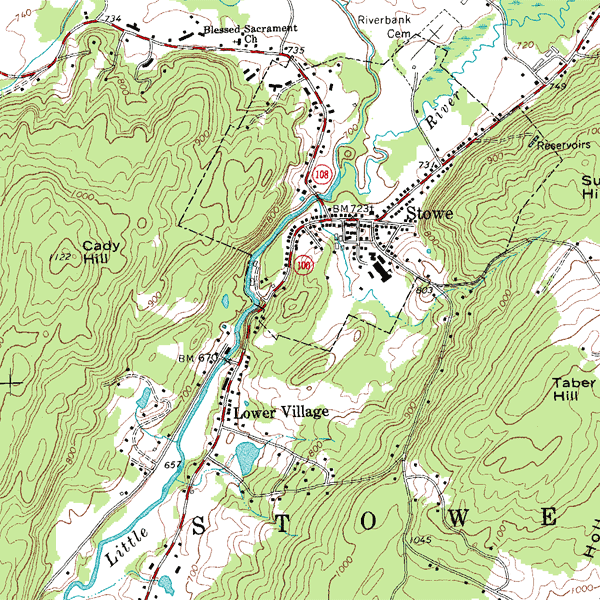United States Geological Survey (Public Domain)

We can see how useful it is to draw distinctions between groups in data, but trying to come up with a efficient way to solve this is not a trivial problem. Let’s simplify this a little more. Say we have a grid of values we want to separate into 2 groups, and to make it simple we will be using be using 0’s and 1’s. If we draw these points as squares it would look something like this.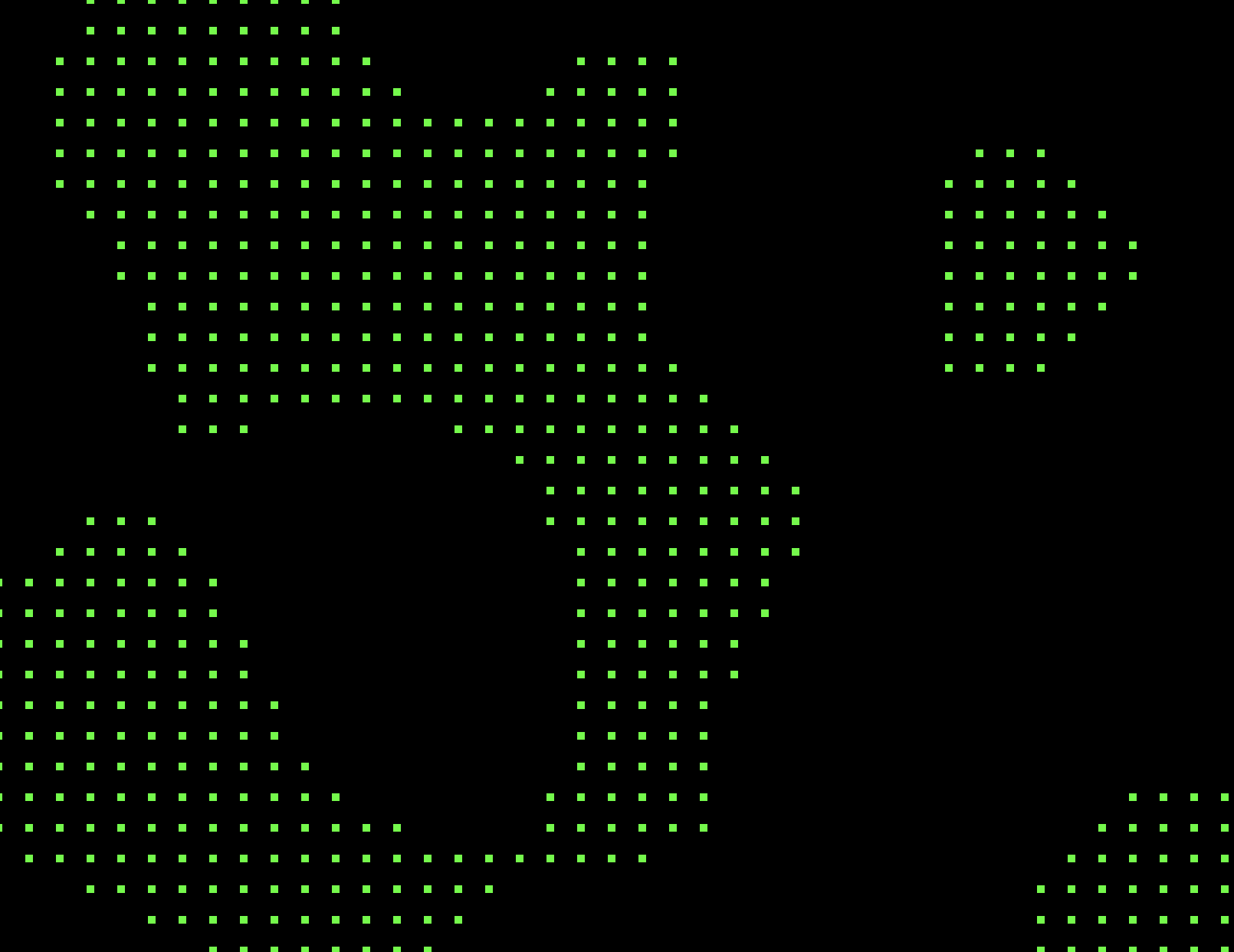Just looking at that image I bet you immediately separate the image into 2 groups, foreground and background. Pretty amazing how our brain does that, but unfortunately computers aren’t as straightforward.
What we want the computer to do the same thing by drawing a line separating the 2 groups. No gaps allowed, and it needs to be done fast enough to see live, like the animation above. This is the goal of the Marching Squares algorithm. If you looked at each group of 4 points, and drew a line according to the this guide, you would come out with a perfect separation between the 2 groups.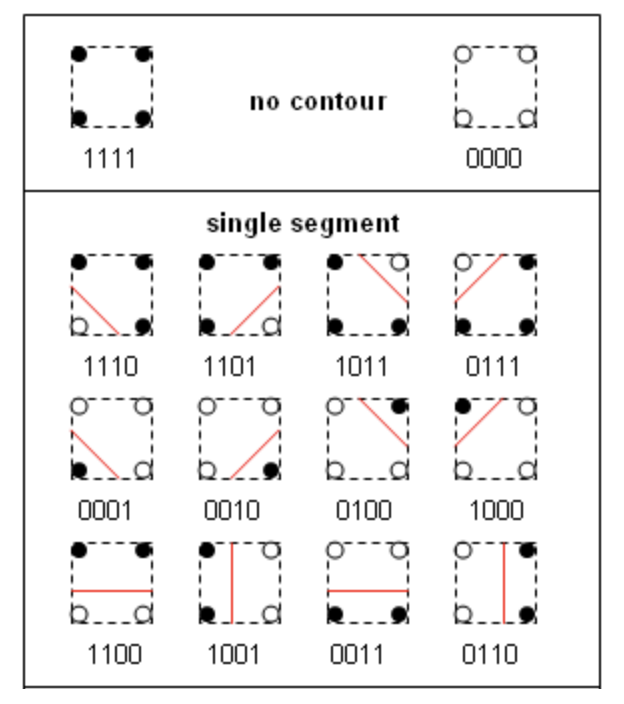Credit: Mikhailfranco

## Prerequisites

This animation was written using p5.js. I would recommend looking at getting started guide if you want to start making animations that you can share on the web! So to get started using this in a project you can simply add this tag to your html.

``````<script src="https://cdn.jsdelivr.net/npm/[email protected]/lib/p5.js"></script>
``````

I will also be using this Perlin noise code to generate our data. Just download it and link it locally in your HTML.

## Set-up

First, we need to set some environment variables.

``````var scalar_array
var SPACE_BETWEEN = 10
var CANVAS_HEIGHT = 500
var CANVAS_WIDTH = 500

generate_noise.seed(Math.random())
function getNoise(x, y) {
let d = new Date()
return generate_noise.perlin3(x / 12, y / 12, d.getTime() / 3000)+1
}
``````

We will store our gid of data in `scalar_array`, and `SPACE_BETWEEN` will be used to change the resolution of the points on the screen. If you have troubles with performance you can try increasing `Space_between`, make sure it can divide into the resolution of the canvas we set later on.
`generate_noise` is a function of the Perlin noise code that I referenced earlier. To use this you need to give it a seed, `Math.random()` will do just fine. Next we need to pass in x, y, and z coordinates to get a value between -1 to 1. We will pass in x and y to the function `getNoise(x,y)` and the current time will be our z coordinate. Just use `generate_noise.perlin3(x, y, z)` from above, and we add 1 to the value so we only get postive values between 0 and 2.

## Creating the Canvas

If you have never used p5.js before there are 2 major functions you will need to know about `Setup()` and `Draw()`.
`Setup()` is called once at the beginning for p5.js. This is where we create the canvas, and set some settings for our animation.

``````function setup() {
canvas = createCanvas(CANVAS_WIDTH, CANVAS_HEIGHT)
canvas.parent('container')
frameRate(30)
loop()
}
``````

`createCanvas(x, y)` will create a canvas of the specified size. `canvas.parent('#id')` sets the canvas tag to be a child of an HTML tag with that ID. Put this HTML tag in you page and the `canvas` will appear there. NOTE! Make sure to link your script below the container tag, otherwise you will get some errors.

``````<div id="container"></div>
``````

`frameRate(FPS)` tells p5.js how many frames a second we want to render, you can also lower this number if you are getting poor performance. And we won’t draw more than one frame unless we specify `loop()` as well.

## Draw!

Alright now for the fun stuff! The `draw()` function is repeatedly called, so we can put our main logic and call to draw things in the canvas here.

``````function draw() {
clear()
background(0)
}
``````

Ok, now we run our code all we get is a blank canvas. Let’s change that!

## Get to the Point

Let’s start getting some points on the screen. First, lets add one more line of code to the beginning of `setup()`

``````scalar_array = Array(CANVAS_WIDTH / SPACE_BETWEEN + 1).fill(0).map(() =>
Array(CANVAS_HEIGHT / SPACE_BETWEEN + 1).fill(0))
``````

This creates a 2d array for `scalar_array` based off our environment variables we made earlier. New we can iterate over each entry in the array to generate a point on the screen.
Now add this next piece of code to the end of `draw()`

``````let square_size = Math.floor(SPACE_BETWEEN / 3)

// Main Loop
for(let x = 0; x < scalar_array.length; x++){
for(let y = 0; y < scalar_array[x].length; y++){
// Create a point object for entry in the array
// TODO: This could be done a lot more efficiently
scalar_array[x][y] = {
data: Math.floor(getNoise(x,y)),
xpos: x * SPACE_BETWEEN,
ypos: y * SPACE_BETWEEN,
x: x,
y: y,
}

// If point is active draw a square
fill(0, 255, 0)
if(scalar_array[x][y].data !== 0){
square(scalar_array[x][y].xpos - square_size / 2, scalar_array[x][y].ypos - square_size / 2, square_size)
}

// WE WILL BE ADDING MORE CODE HERE LATER
}
}
``````

When I mention adding more code to the main loop later, put it where that comment is.
Ok this a bit of to get though, but bear with me. `square_size` is pretty self-explanatory. The Main Loop integrates though each entry in the `scalar_array`. Then we assign each position an object. The most important thing to notice here is the `.data` info. Here we are getting our random value and rounding it down to 0 or 1. Technically we could get 2 as well, but it won’t cause any problems so we will ignore that.
The next important part is where we actually draw the squares. `fill(r,g,b)` sets the color we want to use to fill our squares. The `square(x, y, SIZE)` lets a draw a square on the canvas. It draws the the top left corner at the x and y coordinate we pass to the function, so we will move that by half of the size of the square to center it.
You’re code should draw a canvas similar to the photo from before.

## Drawing a Line in the… Square?

Now for the hard part. We need to come up with an algorithm to draw the lines for each square. We are going to define a square as a point and it’s neighbors up 1 position, left 1 position, and both.

Since any point at the very top or very left of the canvas won’t have any of those neighbors lets add this statement to our main loop before we start adding code to it.

``````if(x > 0 && y > 0){
// More here later
}
``````

We could write a case for every combination of points in a square, and for each case draw a different line. However, I’m lazy so I tried to do the same thing in as little lines of code as possible. Let’s see how this goes.
Now that we are only checking the correct position we can now collect all the points of the square into one array. After we do that, we can filter that array down to just the points are active so we can know how many there are.
Put this inside the if statement block.

``````let current_square = [scalar_array[x - 1][y - 1], scalar_array[x][y - 1], scalar_array[x][y], scalar_array[x - 1][y]]
let active_corners = current_square.filter(point => point.data >= 1)
``````

### One Point Active

Let’s break this problem down step by step. We will start with squares that only have one active point.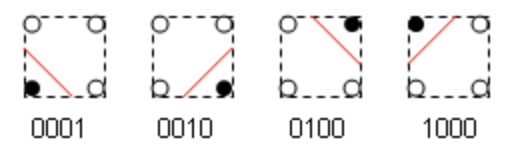If you look at the image you might notice a pattern. A line is just a connection of 2 vectors on the canvas. If you look at the diagram you will notice whenever one of the top two corners are active, one of the vectors is in the top middle of the square. The opposite is true. The same pattern arises when you look at the left vs. the right. Since there is a pattern with a little bit of Math we handle this with a few lines of code. Add this function to the bottom of your Javascript code.

``````function onePointActive(point, x, y){
noFill()
stroke(color(0,255,0))
let xval = point.x - x
let yval = point.y - y
let line_vector = {
x1: x*SPACE_BETWEEN - SPACE_BETWEEN / 2,
y1: (y + yval) * SPACE_BETWEEN,
x2: (x + xval) * SPACE_BETWEEN,
y2: y*SPACE_BETWEEN - SPACE_BETWEEN / 2,
}
line(line_vector.x1, line_vector.y1, line_vector.x2, line_vector.y2)
}
``````

`noFill()`, `stroke()`, and `color()` are all most p5.js functions, if you need to read more on them I’ve provided links.
First, we need to know what corner is active for the current square. This is the purpose of `xval` and `yval`. `xval` will be 0 if the corner in on the bottom of the square, or -1 if it is on the top. `yval` is similar in where it will be 0 if the active corner is on the right or -1 if it is on the left.
We then use these values to make 2 vectors. `x1` and `y1` make up the vector on the top or bottom the square. `x2` and `y2` make up the left or right vector. Now we plug that into the `line()` function and we are good to go!
Now we need to pass a point to this function each time we only have one active point. Add this to our if block from earlier.

``````if(active_corners.length === 1){
onePointActive(active_corners, x, y)
}
``````

You should see an output similar to this now.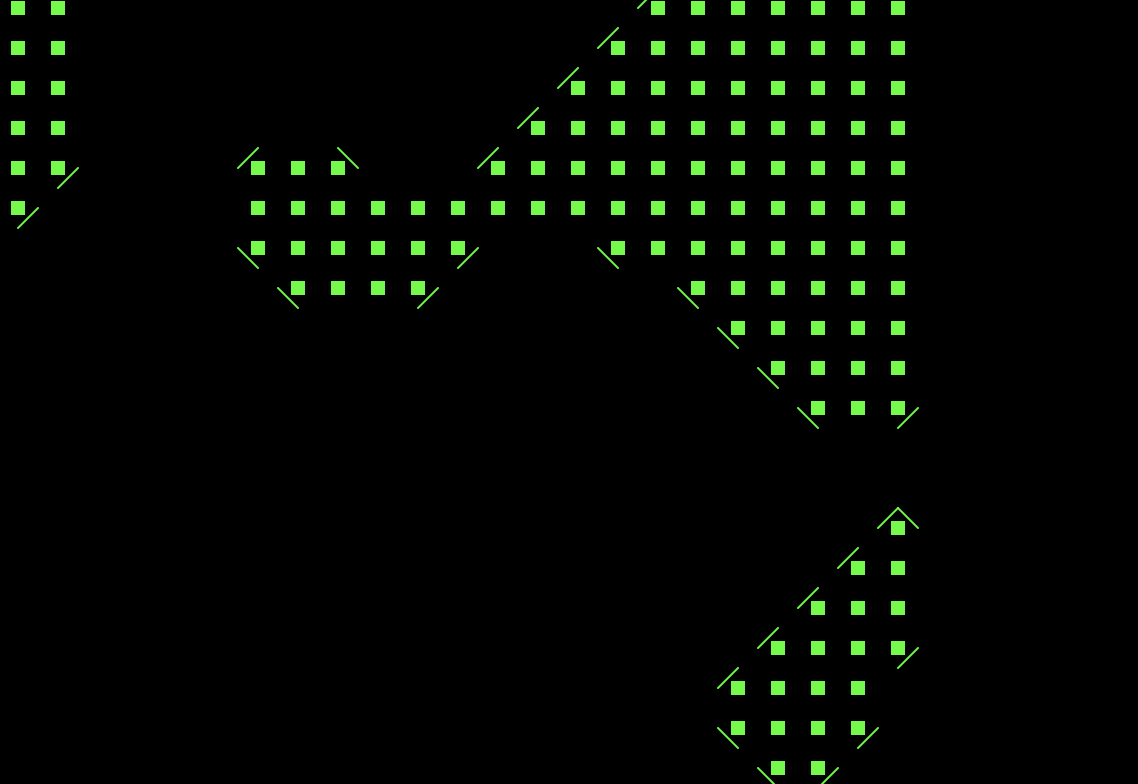### Two Points Active

So new lets figure out what to do if we have 2 points active on out square. We will go ahead put this right after the last if statement we made.

``````else if(active_corners.length === 2){
twoPointsActive(active_corners, x, y)
}
``````

Let’s take a peek at the our guide again.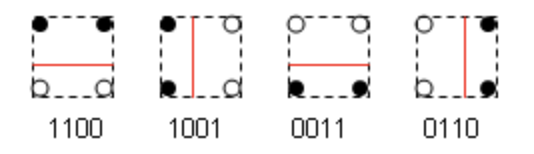This one look even easier. If both points are on the left or right, we draw a vertical line. If both points are both are on the top or bottom, we draw a horizontal line.
Go ahead and slap this function below the `onePointActive` function.

``````function twoPointsActive(active_corners, x, y){
noFill()
stroke(color(0, 255, 0))
// If points are complements treat them both as solo points
if (active_corners.x === active_corners.x){
xval = x * SPACE_BETWEEN - SPACE_BETWEEN / 2
line(xval, y * SPACE_BETWEEN, xval, (y - 1) * SPACE_BETWEEN)
}
else if (active_corners.y === active_corners.y){
yval = y * SPACE_BETWEEN - SPACE_BETWEEN / 2
line(x * SPACE_BETWEEN, yval, (x - 1) * SPACE_BETWEEN, yval)
}
}
``````

This one isn’t too complicated compared to the last one. We are checking the corners if we should draw a vertical or horizontal line. Then we draw the line.

Now we should be getting something like this.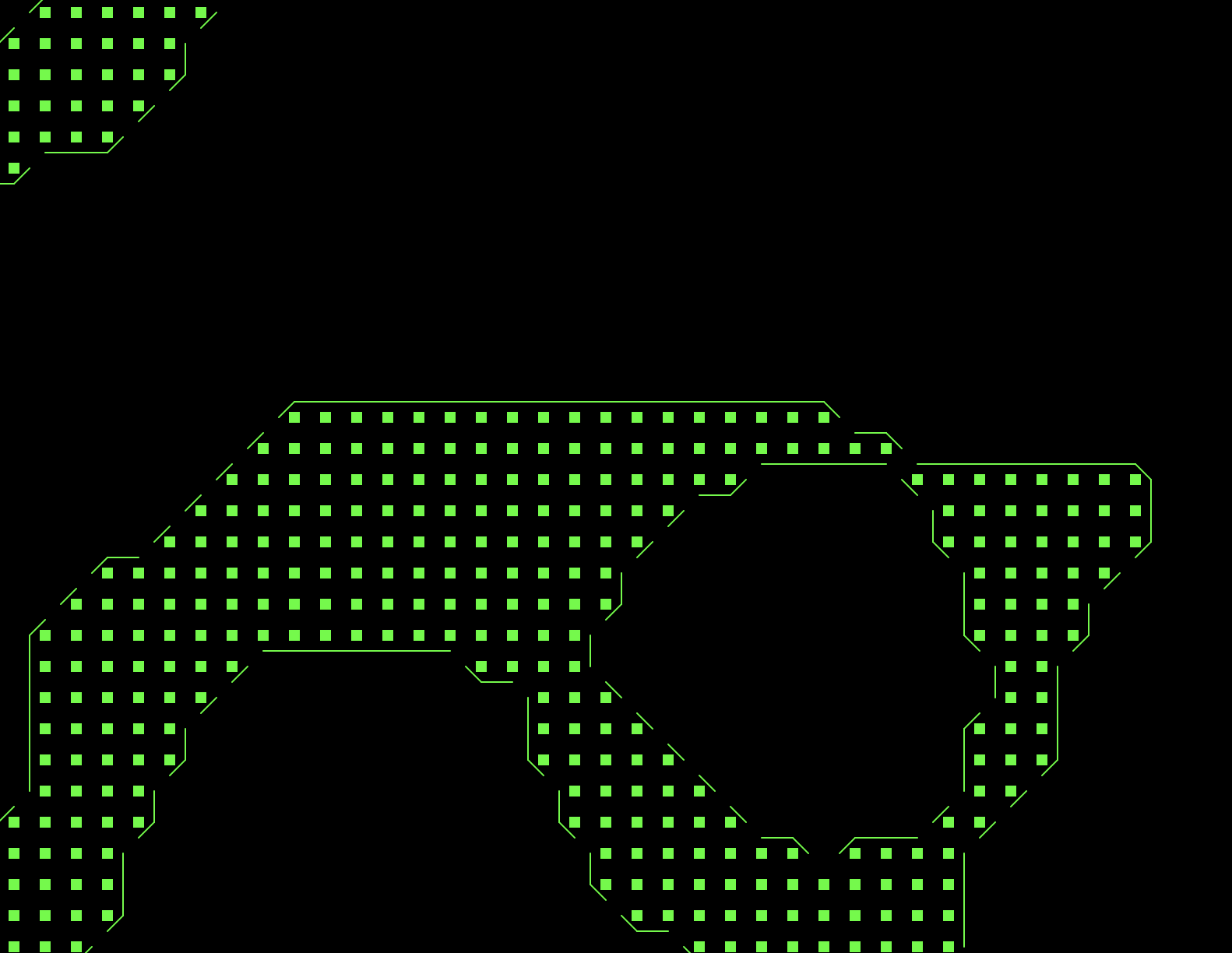### Two Points Active the Sequel

Ok, so that actually wasn’t all the combinations for points. If you noticed what I was skipping over, nice work! Here have a cookie. 🍪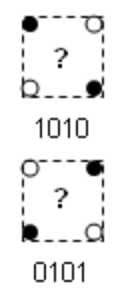There’s actually a couple of ways we could handle opposite active corners. To make things simple lets pretend they each a single active point. It’s a pretty uncommon occurrence anyways. Plus we get to reuse our code from earlier! Add this to the end of `twoPointsActive` function from earlier.

``````else {
onePointActive(active_corners, x, y)
onePointActive(active_corners, x, y)
}
``````

### Three Points Active

Almost there! Now that you know I’m a cheat, you might be able to tell what I’m going to do next.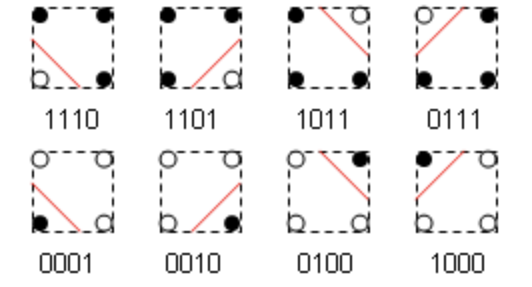Since three points being active is the same line drawn as the opposite one points being active, we can just pass the one point that isn’t active to our `onePointActive` and get the desired result. Add this final chunk of code to our if block at the end of if statment in `draw()` and we will be good to go.

``````else if(active_corners.length === 3){
let inactive_corners = current_square.filter(point => point.data < 1)
onePointActive(inactive_corners, x, y)
}
``````

Here we filter out the active corners instead and pass the one inactive corner to our function.

## End

And that’s it! You should have the complete animation up and running now. If you want to see a couple of extras like resizing the canvas or pausing the animation when it is scrolled out of view checkout the full source code on github below!

You can find the source code for this project on my Github.
If you enjoyed this post leave it a star!

The algorithm used to generate the random data in the animation was written by Joseph Gentle